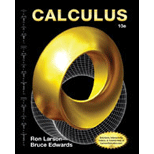# The volume of the solid generated when the region bounded by the curve, y = e − x 2 , y = 0 , x = 0 and x = b , ( b &gt; 0 ) is revolved about the y -axis for b = 1 .### Calculus

10th Edition
Ron Larson + 1 other
Publisher: Cengage Learning
ISBN: 9781285057095### Calculus

10th Edition
Ron Larson + 1 other
Publisher: Cengage Learning
ISBN: 9781285057095

#### Solutions

Chapter 8.1, Problem 87E

(a)

To determine

## To calculate: The volume of the solid generated when the region bounded by the curve, y=e−x2,y=0,x=0 and x=b,(b>0) is revolved about the y-axis for b=1.

(b)

To determine

### Want to see the full answer?

Check out a sample textbook solution.

### Want to see this answer and more?

Experts are waiting 24/7 to provide step-by-step solutions in as fast as 30 minutes!*

*Response times may vary by subject and question complexity. Median response time is 34 minutes for paid subscribers and may be longer for promotional offers.